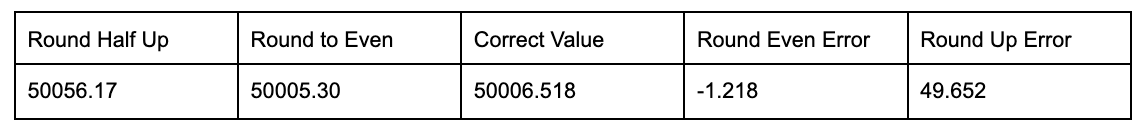##### PostgreSQL and Financial Calculations - Part FiveThe fifth and last in a series of blogs covering common mistakes in Database and Application designs for financial calculations.

## Method of Rounding:

There are many methods of rounding

1. Half Round Up
2. Half Round Down
3. Round Towards Zero
4. Round Away from Zero
5. Round Half To Even
6. Round Half To Odd
7. Random Round

The built-in method of rounding in PostgreSQL is Half Round Up. Unfortunately, it is not the best approach, as it is biased to a higher value. Being biased to a higher value is a well understood problem and why there are so many rounding methods to choose from. To avoid the biased results, the oldest and most common rounding method used is Round Half to Even (commonly referred to as convergent rounding, statistician's rounding, Dutch rounding, Gaussian rounding, or banker’s rounding).

## Consider 5:

`CREATE SCHEMA ol_code;`
`CREATE OR REPLACE FUNCTION ol_code.round(val numeric, prec integer default 0)`
`   RETURNS numeric`
`LANGUAGE 'plpgsql'`
`COST 1`
`STRICT PARALLEL SAFE`
`as \$\$`
`DECLARE`
`_last_digit numeric = TRUNC(ABS((val * (10::numeric^prec) %1::numeric )),1);`
`BEGIN`
`IF _last_digit = 0.5 THEN  --the digit being rounded is 5 `
`-- lets find out if the leading digit is even or odd  `
`IF TRUNC(ABS(val * (10::numeric^prec))) %2::numeric = 0 THEN`
`RETURN trunc(val::numeric,prec);`
`END IF ;`
`END IF ;`
`IF val > 0.0 AND _last_digit >= 0.5 THEN`
`RETURN  trunc(val::numeric + (1/ (10::numeric^prec)), prec) ;`
`ELSEIF  val > 0.0 AND _last_digit < 0.5 THEN`
`RETURN trunc(val::numeric, prec);`
`ELSEIF val < 0.0 AND _last_digit >= 0.5 THEN`
`RETURN  trunc(val::numeric - (1/ (10::numeric^prec)), prec) ;`
`ELSE`
`RETURN  trunc(val::numeric, prec);`
`END IF;`
`END ;`
`\$\$;`
`WITH cc as (select random()::numeric as random  from generate_series(0,100000) )`
`select  sum(pg_catalog.round(random,2)) round_half_up, `
`sum(ol_code.round(random,2)) as round_to_even,`
`sum(trunc(random,3)) correct_value ,  `
`sum(ol_code.round(random,2)) - sum(trunc(random,3)) round_even_error,`
`sum(pg_catalog.round(random,2)) - sum(trunc(random,3)) round_up_error`
`from cc`0.1% error Round Half Up vs 0.0025% error Round To Even

As we can see above, the rounding method we are all taught in school creates error biasing the value to the high side compared to banker’s rounding, which we should all be using.

## The solution:

To fix the rounding in PostgreSQL, we need to implement a custom rounding function and overload the default round function by setting the search path like so:

SET search_path to ol_code, pg_catalog, public

This assumes the custom round function is named round(numeric,integer) and placed in the schema ol_code (ol_code is short for overloaded code). This schema is where I place any function overloading the default behavior of PostgreSQL.

There are two well known standards for rounding: ASTM E29 and IEEE 754. Both specify the Round Half to Even method. To maintain the highest level of accuracy, Round Half Up should be replaced with Round to Even, as it is the preferred method.

## Closing Thoughts:

If all the issues discussed in this series were trivial problems we would not have numeric types, independent Math libraries, international standard documents or math papers to address the problems.

Rounding and precision math errors can not be stopped, only contained and limited. It is up to us to use the appropriate tools and techniques to contain the error, limiting the havoc it will create.

These are solved problems, we just need to use the solution.